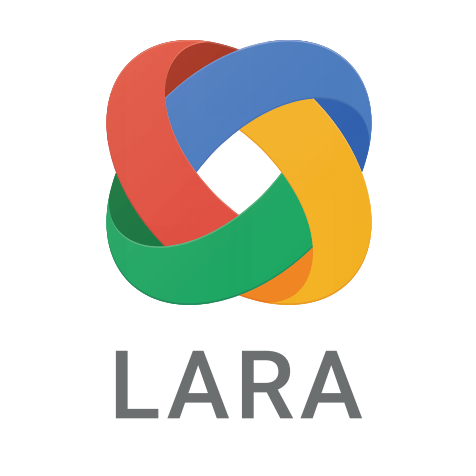# Private ML

Machine learning over encrypted data using homomorphic encryptionUniversity of Campinas 40,000 USD from Google 2015-2017
Principal Investigator

Homomorphic encryption has a very important role in Cloud Computing because it allows the data to be processed without being revealed. It means that users of cloud services can have both the convenience and the privacy by encrypting and then submitting their data. However, Fully Homomorphic Encryption schemes, that would allow the cloud to execute arbitrary functions over encrypted data, are still not practical. Hence, researchers have studied also how to use Somewhat Homomorphic Encryption to solve several problems, like calculating logistical regression or running classification algorithms over encrypted data. This project will develop and implement homomorphic versions of two algorithms widely used in machine learning that can be evaluated over encrypted data using somewhat homomorphic encryption schemes: Principal Component Analysis (PCA) and k Nearest Neighbors (k-NN).